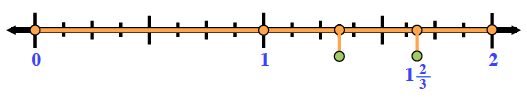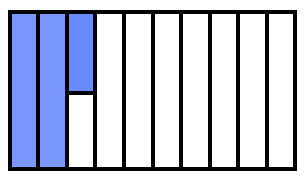### Home > ACC6 > Chapter 6 Unit 6 > Lesson CC1: 6.2.4 > Problem6-108

6-108.

Represent each of the following numbers two ways: by drawing an area model and by using a number line.

1. $1\frac { 2 } { 3 }$

• Use the number line below to help you draw a diagram.1. $125\%$